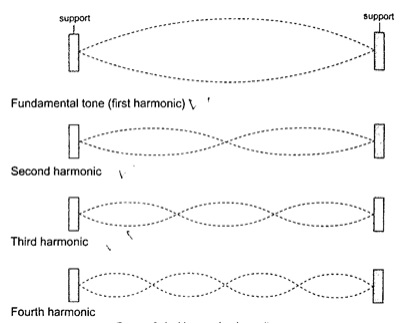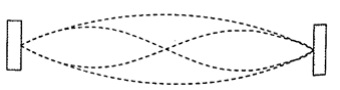# Sound

### Guest Account

Attempt Form Two Physics Questions
Guest Account

# Sound

- Sound is an important example of a longitudinal wave which is composed of both compressions and rarefactions.
- The frequency range in which compressional disturbances occur is called the sonic spectrum and is very large.
- Compressional waves with frequencies above and below the audio range are called ultrasonic and infrasonic frequencies respectively.

### Nature and transmission of sound waves

- Sound waves require a material medium for transmission from one point to another.
- Sound waves therefore cannot be transmitted in a vacuum.
- The rate of transmission diminishes as you move from solids, to liquids then to gases.

### Characteristics of sound waves

1. Intensity and loudness – intensity of sound refers to the rate of flow of energy through an area. The loudness of a sound generally varies with the intensity of sound. The frequency of sound waves determines their intensity while the amplitude determines their loudness.
2. Frequency and pitch – pitch refers to the sharpness of a sound and is determined by its frequency.
3. Fundamental tones and harmonics – fundamental frequency is the vibration in a wire which has the lowest possible frequency. The fundamental frequency and the tones with the frequencies that are whole multiples of the fundamental are called harmonics. The fundamental frequency is also the first harmonic. The number of segments vibrating in a string depends on the point at which the string is plucked.### Quality of sound or timbre

– quality of sound can be improved by adding the second harmonic to the fundamental frequency hence the quality depends on the number of harmonics produced simultaneously and their relative intensities.## Echo

- Echoes are produced by reflection of sound waves from hard surfaces such as a wall or a cliff.
- To determine the velocity of sound ‘v’ between two successive claps is given as v = distance from the reflecting surface / half the time taken between two successive claps.
Hence; speed ‘v’= distance / time = m/s.

Example 1
A boy strikes a railway line (steel) with a hammer. If the speed of sound in steel is 5,200 m/s, determine the time taken for the sound to reach another boy 2.3 km down the railway line with his ear on the rail.
Solution
Time taken = distance / speed = 2300 / 5200 = 0.44 seconds.

### Factors affecting the speed of sound

a) Temperature – speed increases with increase in temperature especially gases since the change in liquids and solids is small it can be neglected.
b) Nature of transmitting medium – different substances transmit sound waves at various speeds as shown below;
• Air – 346 m/s
• Hydrogen – 1339 m/s
• Water – 1498 m/s
• Aluminium – 5,000 m/s
• Iron – 5,200 m/s
• lass – 4,540 m/s
Example 1
A girl claps her hands once at a distance 250 m from a vertical cliff. If the temperature in the surrounding is 50 C, how long does it take for her to hear the echo?
Solution
Distance travelled = 250 × 2 = 500 m.
Speed of sound = (331.5 m/s at 00) the speed in air increases at about 0.6 m/s per 0°C.
Therefore speed at 50 C = 331.5 + (0.6 × 5) = 334.5 m/s.
Time taken = distance / speed = 500 / 334.5 = 1.5 seconds.